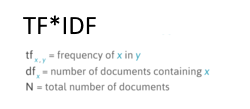# TF*IDF in C# .NET for Machine Learning - Term Frequency Inverse Document Frequency

## Introduction

During a recent machine learning competition, I was searching for an example of working code in C# .NET that performed a term frequency inverse document frequency TF*IDF transformation on a set of documents. For those not familiar, TF*IDF is a numerical value that indicates how important a word is within a document, compared with a larger set of documents (or corpus). It’s used in search engine ranking algorithms, natural language processing, machine learning, and various statistics algorithms.

In this particular case, I required a method for extracting features from a list of search result documents. That is, given a large set of strings, the goal was to determine some type of unique significance amongst each document, thereby converting each document into a vector (an array of values). The resulting values would then be fed into a machine learning algorithm, which could hopefully predict accurate results for future unknown documents.

The TF*IDF algorithm is fairly straightforward, as described below. For such a relatively simple mathematical formula, I had hoped there would be a library available for easy importing into a C# .NET project. After a bit of searching, I decided to create my own TF*IDF transformation class, modeled after the Python scikit-learn library’s method, TfidfVectorizer(), which takes a list of documents and returns a matrix (multidimensional array of doubles) of TF*IDF values.

This TF*IDF implementation in C# .NET may be used to easily convert a list of documents into their corresponding TF*IDF values. The class takes care of tokenizing the documents, skipping stop words, stemming, building a vocabulary, and finally transforming the strings into their associated TFIDF values.

## Overview of TF*IDF

TF*IDF is the shorthand description for Term Frequency * Inverse Document Frequency. It essentially consists of two simple formulas for judging the importance of words within a document, against a larger set of documents (also called the corpus). A worked example is shown below. For a detailed walkthrough of how to calculate TFIDF for a string, see here (towards bottom of page).## Term Frequency

Term frequency (TF) is a count of how many times a particular term appears within a document. It is calculated by simply counting the number of times the word is found in the target document. For example, assume we’re calculating for the term “sun”:

## Inverse Document Frequency

Inverse document frequency (IDF) is a count of how many documents in the entire corpus contain the term. Suppose we have a corpus of 100 documents with 20 of those documents containing the word “sun”.

Since the corpus might not contain any documents matching the word, it’s common to add 1 to the matching document count to avoid a division by zero error.

The final calculation for TF*IDF is to simply multiply TF * IDF.

## Normalization

After obtaining the TF*IDF values for a list of documents, it’s common to normalize the values. This can be particularly helpful when using the values for machine learning techniques, such as feeding them as inputs into a logistic regression or SVM algorithm. A common normalization algorithm used with TF*IDF is the L2 normalization process.

## TF*IDF C# .NET Class

The full source code for the class may be found on GitHub. The class takes an array of strings (documents) as input and returns a multidimensional array of doubles (vectors). Each vector corresponds to an encoded document and its associated TF*IDF values against the corpus vocabulary.

An example of running the algorithm:

In the example above, the vocabulary consists of 5 terms (resulting in 2 rows and 5 columns in the matrix).

The class also includes an optional method for normalizing the resulting vectors, using L2-norm: Xi = Xi / Sqrt(X0^2 + X1^2 + .. + Xn^2). Applying normalization to the above example produces the following result:

## Building the Vocabulary

The above method first parses the list of documents to tokenize and extract the list of relevant words. The method GetVocabulary() parses the documents and builds a vocabulary for the corpus. Once the vocabulary is ready, we can calculate the IDF for each term in the vocabulary. The calculation requires us to know the target term and the number of documents containing the term.